# Linear regression with straight lines

Linear regression is a widely used mathematical technique in which a certain function (often a straight line or polynomial) is used to fit a set of data or raw measurements, showing a correlation between 2 or more variables. The idea is that we choose a certain function which represents a measure of the error between the “line of best fit” and the actual data, and then we use techniques from Calculus to minimize that error by choosing the most optimum parameters for that line of best fit. In this article, I will walk the reader through the mathematics of linear regression with straight lines using the technique of least squares analysis (LSA).

*Note: it is assumed the reader is familiar with some multivariable calculus (e.g. partial derivatives) and linear algebra.

# Mathematical derivation

We begin our discussion by the most common and basic of all regression types: straight line regression. As its name suggests, given a data set S(x), our goal is to find the equation of a straight line y(x) which minimises the distance between each point on the line and the data set S(x). In general, the equation of a straight line is written as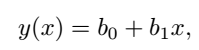Equation 1: line of best fit

where the parameters b0 and b1 are constants to be determined. In order to calculate these parameters, we create a function that represents the sum of the squared error E for each point in y(x) and the data set S(x)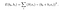Equation 2: sum squared error

The idea is then to to minimise the function E(b0, b1) in terms of all of its parameters, by finding all first partial derivatives, namely

Because the turning points occur when these derivatives are equal to zero, we can write the equations as

Now, separating terms into individual sums yields

These equations can be written in matrix form as

Leading to the solution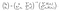Equation 3: matrix solution for the coefficients b0 and b1

which gives the values of b0 and b1 which minimize E(b0, b1) for the given data set S(x). Because the matrices are small, we can analytically find the inverse as

leading to the closed-form expressions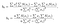Equation 4 (a) - (b): analytic solutions for b0 and b1

# Implementation in MATLAB

We will now implement this linear regression process in MATLAB (although the code used here can easily be adapted to any programming language of your choice).

The code has the following steps:

1. We create an array x and a random data-set S(x) with a constant slope, but we add a random number at each point x so that not all the points fall on the straight line
2. We build up the 2x2 coefficient matrix containing the sum terms of x and x², call it matrix A
3. The vector on the right of Eq(3) is called RHS and contains sums of terms involving S(x) and x
4. Solve the linear system using b = A\RHS (left-hand matrix division in MATLAB, equivalent to taking the inverse of A and multiplying by RHS)

%%%%%%%%%%%%%%%%%%%%%%%%%%%%%%%%%%%%%%%

% Least-Squares-Analysis (LSA) for linear fit

%

% Description: generates a random data-set around a linear curve with

% preset gradient and then uses LSA matrix methods to find the coefficients

% of the line of best-fit, and overlays the fit-line to the dataset:

% Equation → y = b0 + b1*x

%

% Made by: Oscar A. Nieves

% Made in: 2019

%%%%%%%%%%%%%%%%%%%%%%%%%%%%%%%%%%%%%%%

clear all; close all; clc;

% Generate dataset

x = 0:0.1:10;

S = 2*x + randn(size(x));

% Use LSA to find line of best fit

n = length(S);

sum_x = sum(x);

sum_x2 = sum(x.²);

sum_S = sum(S);

sum_xS = sum(x.*S);

A = [ [n, sum_x]; …

[sum_x, sum_x2] ];

RHS = [sum_S, sum_xS].’;

b = A\RHS;

b0 = b(1);

b1 = b(2);

% Fit straight line to data and generate plots

y = b0 + b1*x;

figure(1);

set(gcf,’color’,’w’);

scatter(x,S); hold on;

plot(x,y,’r’,’LineWidth’,3); hold off;

legend(‘Raw Data’,’Fit-line’); legend boxoff;

legend(‘Location’,’northwest’);

title([‘b_0 = ‘ num2str(round(b0,2)) ‘, b_1 = ‘ num2str(round(b1,2))]);

xlabel(‘x’);

ylabel(‘y’);

axis tight;

set(gca,’FontSize’,20);

%%%%%%%%%%%%%%%%%%%%%%%%%%%%%%%%%%%%%%%

The result for a single run of this code is shown here: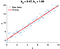Figure 1: LSA linear regression of a straight line

As we can see, the red line falls in between the raw data points in such a way so as to minimize the square distance between the line of best fit and the data. Optimum parameters b0 and b1 are shown at the top of Figure 1.

Oscar is a physicist, educator and STEM enthusiast. He is currently finishing a PhD in Theoretical Physics with a focus on photonics and stochastic dynamics.

## More from Oscar Nieves

Oscar is a physicist, educator and STEM enthusiast. He is currently finishing a PhD in Theoretical Physics with a focus on photonics and stochastic dynamics.